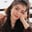Related Tags

dot
dataframe
pandas
python
communitycreator

# DataFrame.dot() method in pandasFouzia Bashir

## Overview

The dot() method is used to compute the dot product between DataFrames or Series. DataFrame.dot() from DataFrame class is used to take the dot product of two DataFrames or DataFrame and Series.

Note: A dot() works like a mul() function, but instead of returning multiplication separately, it returns the sum of multiplied values at each row or index.

### Syntax

# Signature
DataFrame.dot(other)

### Parameters

It takes the following argument value:

• other: Another object of DataFrame or Series or array-like to compute dot product.

### Return value

It returns either Series or DataFrame.

• If other is Series, it returns series after simple matrix dot product.
• If other is DataFrame, it returns DataFrame after dot product.

### Code

Let's discuss different scenarios with some coding examples. Here we have listed some commonly used cases:

1. Only DataFrames
2. DataFrame and Series

### Only DataFrames

In this code, we'll take two DataFrame of the same dimensions and evaluate their dot product.

# importing pandas module as alias pd
import pandas as pd
# Creating a DataFrame
df1 = pd.DataFrame([[0, 1, -2, -1],
[0, 1, None, 1],
[1, 3, 1, -1],
[1, 1, 6, 1]])
# Creating a DataFrame
df2 = pd.DataFrame([[5, 3, 6, 4],
[11, 3, 4, 3],
[4, 3, 8, 3],
[5, None, 2, 8]])
# Calculating dot product
print(df1.dot(df2))

### Explanation

• Lines 4 to 7: We create a numeric DataFrame df1 that contains four observations.Number of rows
• Lines 9 to 12: We create a numeric DataFrame df2 that contains four observations.
• Line 14: We invoke df1.dot(df2) to evaluate dot product in return.

### DataFrame and Series

In this code snippet, we'll create a pandas DataFrame and Series object to calculate their dot product.

# importing pandas as pd
import pandas as pd
# Creating the dataframe
df = pd.DataFrame([[5, 3, 6, 4],
[11, None, 4, 3],
[4, 3, 8, None],
[5, 4, 2, 8]])
# Creating a series
series = pd.Series([1, 1, 2, 1])
# Print the dot product between DataFrame and Series
print(df.dot(series))

### Explanation

• Lines 4 to 7: We create a numeric DataFrame df that contains four observations.
• Line 9: We instantiate Series to get Pandas series object of four values.
• Line 11: We invoke df.dot(series) to get dot product between DataFrame and series.

RELATED TAGS

dot
dataframe
pandas
python
communitycreator

CONTRIBUTORFouzia Bashir
RELATED COURSES

View all Courses

Keep Exploring

Learn in-demand tech skills in half the time